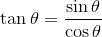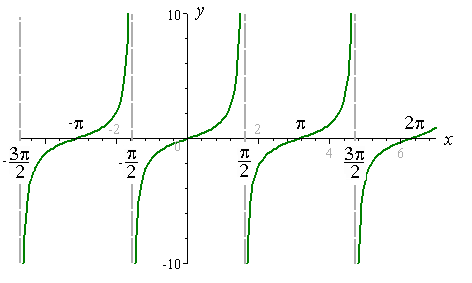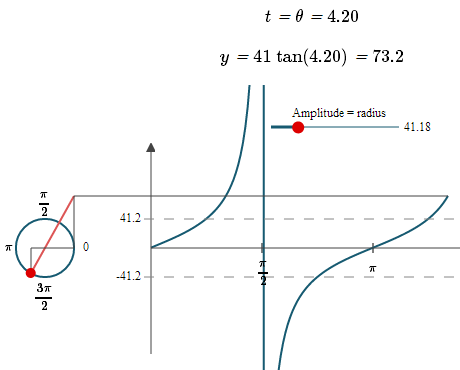Search IntMath
Close

# Updated Graphs of tan, cot, sec and csc

By Murray Bourne, 30 Jul 2014

One of the most popular pages in IntMath is the one explaining Graphs of tan, cot, sec and csc.

To make the page more mobile-device friendly, I updated it so now the interactive graphs use JSXGraph, rather than Flash.

The page explains how to sketch these trigonometric curves, mostly by considering the denominator (the bottom) of the function expression.

Each of the curves tan θ, cot θ, sec θ and csc θ has a discontinuity, or "gap" in the curve. For example, tan θ is defined by:Since cos θ has value 0 at θ = ... −3π/2 −π/2, π/2, 3π/2, 5π/2,..., it means tan θ will not exist for those values of θ. The result is there will be "gaps" in the curve at those points.

Either side of those gaps, the curve heads off to plus or minus infinity. Here is the graph of y = tan θ:The page has animations that demonstrate where the values of the curves come from.

Here's a screen shot of one of the animations:On a tablet, the animations may run a little slow. After all, we're asking the processor to perform a lot of calculations! Alo, the screen gets re-drawn multiple times during the animation, which also slows things down.

As a result, you can only animate one of the graphs at a time.

Graphs of tan, cot, sec and csc

See the 1 Comment below.

### Comment Preview

HTML: You can use simple tags like <b>, <a href="...">, etc.

To enter math, you can can either:

1. Use simple calculator-like input in the following format (surround your math in backticks, or qq on tablet or phone):
a^2 = sqrt(b^2 + c^2)
(See more on ASCIIMath syntax); or
2. Use simple LaTeX in the following format. Surround your math with $$ and $$.
$$\int g dx = \sqrt{\frac{a}{b}}$$
(This is standard simple LaTeX.)

NOTE: You can mix both types of math entry in your comment.